## GPU Code Generation for Blocks from the Deep Neural Networks Library

With GPU Coder™, you can generate optimized code for Simulink® models containing a variety of trained deep learning networks. You can implement deep learning functionality in Simulink by using MATLAB Function blocks or by using blocks from the Deep Neural Networks library. The Deep Neural Networks block library includes:

• Predict (Deep Learning Toolbox) block — Predict responses using the trained network specified through the block parameter. This block enables loading of a pretrained network into the Simulink model from a MAT-file or from a MATLAB® function.

• Image Classifier (Deep Learning Toolbox) block — Classify data using a trained deep learning neural network specified through the block parameter. This block enables loading of a pretrained network into the Simulink model from a MAT-file or from a MATLAB function.

You can configure the code generator to take advantage of the NVIDIA® CUDA® deep neural network library (cuDNN) and TensorRT™ high performance inference libraries for NVIDIA GPUs. The generated code implements the deep convolutional neural network (CNN) by using the architecture, the layers, and parameters that you specify in the network object.

### Example: Classify Images by Using GoogLeNet

GoogLeNet has been trained on over a million images and can classify images into 1000 object categories (such as keyboard, coffee mug, pencil, and animals). The network takes an image as input, and then outputs a label for the object in the image with the probabilities for each of the object categories. This example shows how to perform simulation and generate CUDA code for the pretrained `googlenet` deep convolutional neural network and classify an image. The pretrained networks are available as support packages from the Deep Learning Toolbox™.

```net = googlenet; ```

2. The object `net` contains the `DAGNetwork` object. Use the `analyzeNetwork` function to display an interactive visualization of the network architecture, to detect errors and issues in the network, and to display detailed information about the network layers. The layer information includes the sizes of layer activations and learnable parameters, the total number of learnable parameters, and the sizes of state parameters of recurrent layers.

```analyzeNetwork(net); ```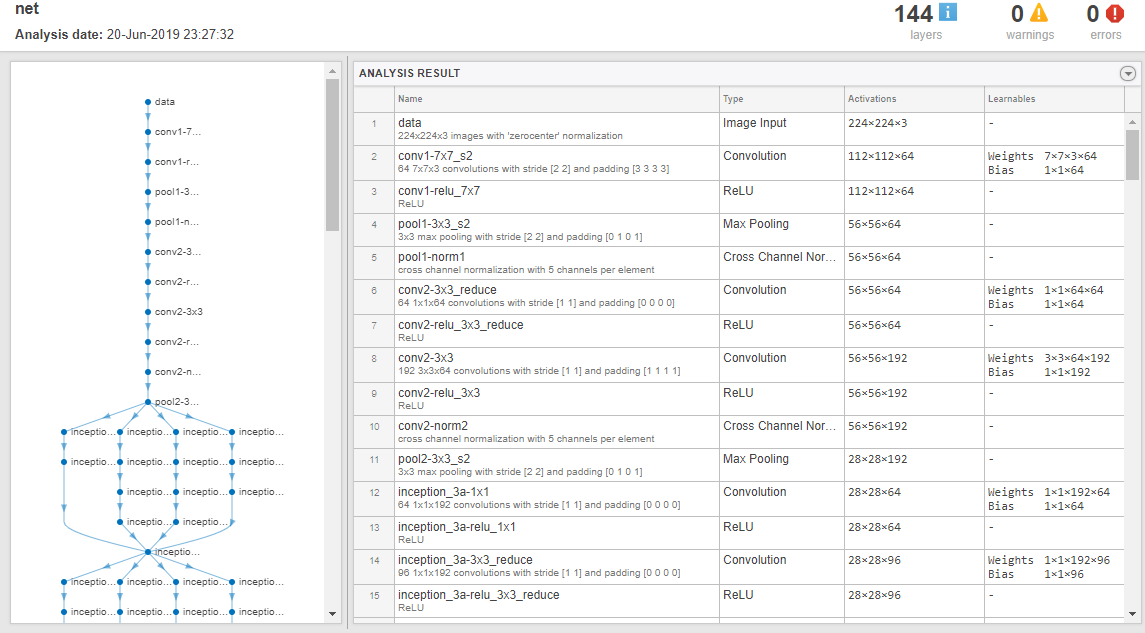3. The image that you want to classify must have the same size as the input size of the network. For GoogLeNet, the size of the `imageInputLayer` is 224-by-224-by-3. The `Classes` property of the output `classificationLayer` contains the names of the classes learned by the network. View 10 random class names out of the total of 1000.

```classNames = net.Layers(end).Classes; numClasses = numel(classNames); disp(classNames(randperm(numClasses,10))) ```
``` 'speedboat' 'window screen' 'isopod' 'wooden spoon' 'lipstick' 'drake' 'hyena' 'dumbbell' 'strawberry' 'custard apple' ```

1. Create a Simulink model and insert a Predict block from the Deep Neural Networks library.

2. Add an Image From File block from the Computer Vision Toolbox™ library and set the `File name` parameter to `peppers.png`. Add a Resize block from the Computer Vision Toolbox library to the model. Set the Specify parameter of the Resize block to ```Number of output rows and columns``` and enter `[224 224]` as the value for Number of output rows and columns. The resize block resizes the input image to that of the input layer of the network. Add a To Workspace to the model and change the variable name to `yPred`.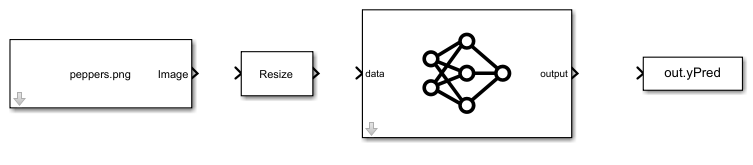3. Open the Block Parameters (subsystem) of the Predict block. Select ```Network from MATLAB function``` for Network and googlenet for MATLAB function.

4. Connect these blocks as shown in the diagram. Save the model as `googlenetModel.slx`.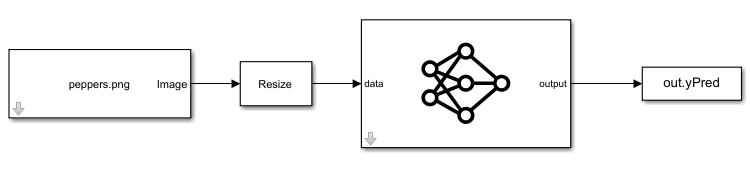### Configure the Model for GPU Acceleration

Model configuration parameters determine the acceleration method used during simulation.

1. Open the Configuration Parameters dialog box. Open the Solver pane. To compile your model for acceleration and generate CUDA code, configure the model to use a fixed-step solver. This table shows the solver configuration for this example.

ParameterSettingEffect on Generated Code
Type`Fixed-step`Maintains a constant (fixed) step size, which is required for code generation
Solver`discrete (no continuous states)`Applies a fixed-step integration technique for computing the state derivative of the model
Fixed-step size`auto`Simulink chooses the step size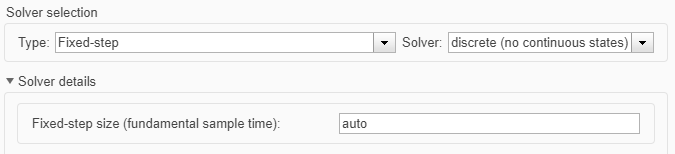2. Select the Simulation Target pane. Set the Language to `C++`.

3. Select GPU acceleration. Options specific to GPU Coder are now visible in the Simulation Target > GPU Acceleration pane. For this example, you can use the default values of these parameters.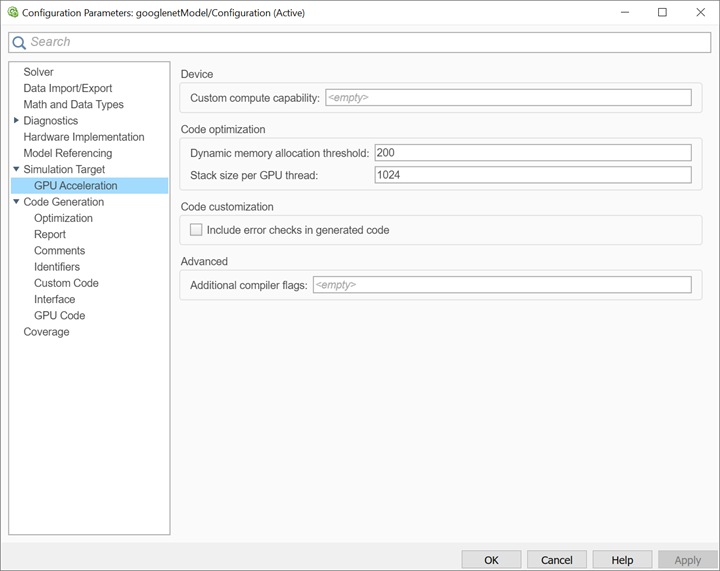4. On the Simulation Target pane, set the Target Library in the Deep learning group to `cuDNN`. You can also select `TensorRT`.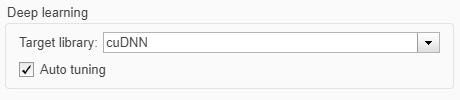5. Click OK to save and close the Configuration Parameters dialog box.

You can use `set_param` to configure the model parameter programmatically in the MATLAB Command Window.

```set_param('googlenetModel','GPUAcceleration','on'); ```

### Build GPU Accelerated Model

1. To build and simulate the GPU accelerated model, select Run on the Simulation tab or use the command:

```out = sim('googlenetModel'); ```

The software first checks to see if CUDA/C++ code was previously compiled for your model. If code was created previously, the software runs the model. If code was not previously built, the software first generates and compiles the CUDA/C++ code, and then runs the model. The code generation tool places the generated code in a subfolder of the working folder called `slprj/_slprj/googlenetModel`.

2. Display the top five predicted labels and their associated probabilities as a histogram. Because the network classifies images into so many object categories, and many categories are similar, it is common to consider the top-five accuracy when evaluating networks. The network classifies the image as a bell pepper with a high probability.

```im = imread('peppers.png'); predict_scores = out.yPred.Data(:,:,1); [scores,indx] = sort(predict_scores,'descend'); topScores = scores(1:5); classNamesTop = classNames(indx(1:5)) h = figure; h.Position(3) = 2*h.Position(3); ax1 = subplot(1,2,1); ax2 = subplot(1,2,2); image(ax1,im); barh(ax2,topScores) xlabel(ax2,'Probability') yticklabels(ax2,classNamesTop(5:-1:1)) ax2.YAxisLocation = 'right'; sgtitle('Top 5 predictions using GoogLeNet') ```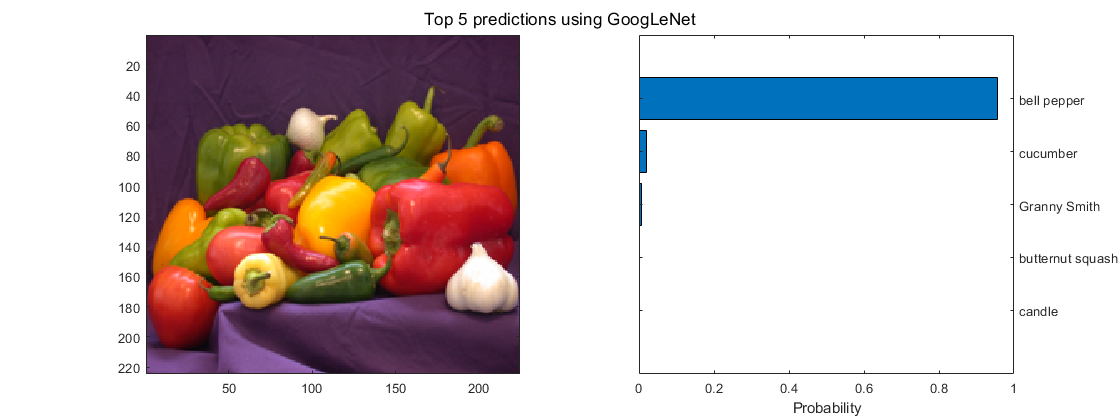### Configure Model for Code Generation

The model configuration parameters provide many options for the code generation and build process.

1. In Configuration Parameters dialog box, select Code Generation pane. Set the System target file to `grt.tlc`.

You can also use the Embedded Coder® target file `ert.tlc`.

2. Set the Language to `C++`.

3. Select Generate GPU code.

4. Select Generate code only.

5. Select the Toolchain. For Linux® platforms, select ```NVIDIA CUDA | gmake (64-bit Linux)```. For Windows® systems, select ```NVIDIA CUDA (w/Microsoft Visual C++ 20XX) | nmake (64-bit windows)```.

6. On the Code Generation > Report pane, select Create code generation report and Open report automatically.

7. On the Code Generation > Interface pane, set the Target Library in the Deep learning group to `cuDNN`. You can also select `TensorRT`.

8. Options specific to GPU Coder are in the Code Generation > GPU Code pane. For this example, you can use the default values of the GPU-specific parameters in Code Generation > GPU Code pane.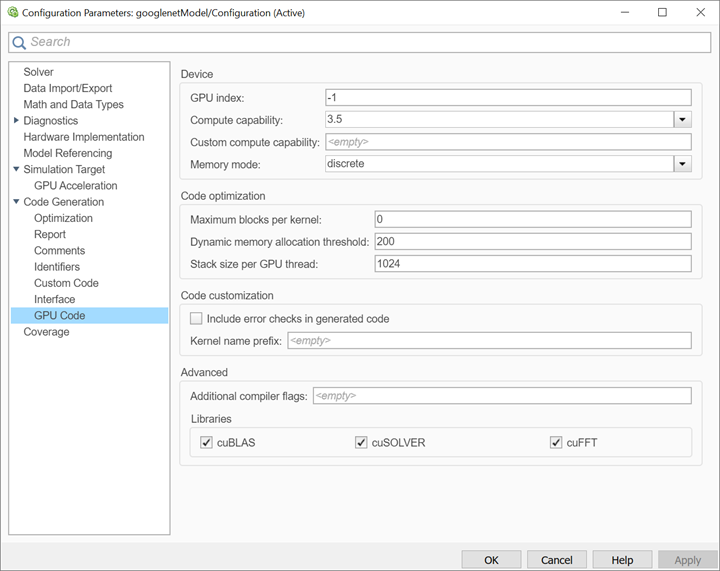9. Click OK to save and close the Configuration Parameters dialog box.

You can also use `set_param` to configure the model parameter programmatically in the MATLAB Command Window.

```set_param('googlenetModel','GenerateGPUCode','CUDA'); ```

### Generate CUDA Code for the Model

2. Generate code.

Messages appear in the Diagnostics Viewer. The code generator produces CUDA source and header files, and an HTML code generation report. The code generator places the files in a build folder, a subfolder named `googlenetModel_grt_rtw` under your current working folder.

### Limitations

• Code generation for a deep learning network with custom layer is not supported in Simulink.

• GPU code generation for MATLAB Function blocks in Stateflow® charts is not supported.

• The code generator does not support all the data types from the MATLAB language. For supported data types, refer to the block documentation.

• For deploying the generated code, it is recommended to use the Generate an example main program option to generate the `ert_main.cu` module. This option requires the Embedded Coder license.

You can also use the `rt_cppclass_main.cpp` static main module provided by MathWorks®. However, the static main file must be modified such that the models class constructor points to the deep learning object. For example,

```static googlenetModelModelClass::DeepLearning_googlenetModel_T googlenetModel_DeepLearning; static googlenetModelModelClass googlenetModel_Obj{ &googlenetModel_DeepLearning}; ```